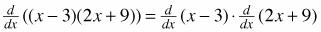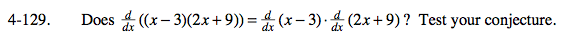### Home > CALC > Chapter 4 > Lesson 4.4.1 > Problem4-129

4-129.

Does? Test your conjecture. Homework Help ✎Evaluate the left-side of the equation first. Be sure to multiply before you differentiate.

Next evaluate the two derivatives on the right side of the equation. Multiply after you differentiate. Do the two answers agree?

In this course, you will discover several shortcuts to help you find derivatives. This is not one of them!